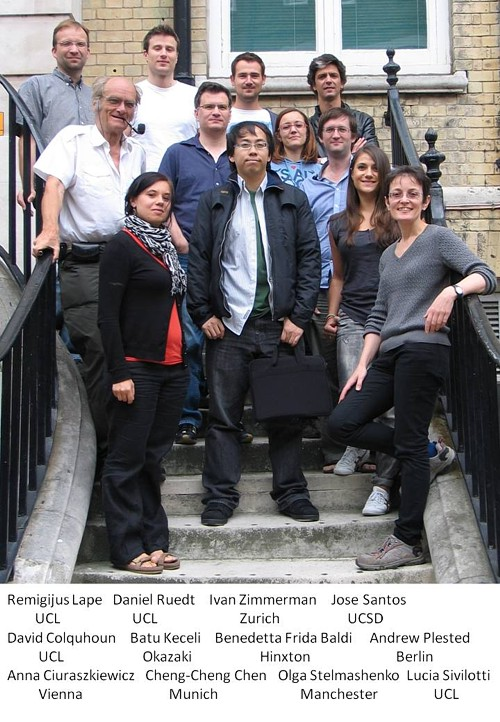# Summer Workshop

### Understanding Ion Channel Currents in Terms of Mechanisms

Analysis and interpretation of single ion channel records and macroscopic currents using matrix methods.

The 2013 course ran from 8 – 12 July, 2013. Course photo here.

This course ran from 2003 to 2013.  A total of 133 students from many countries have done it.  They ranged from graduate students to full professor.

The mornings were devoted to talks.  In the afternoons the attendees worked in their laptops using Mathcad to implement the equations that were presented in the talks and to get numerical results from them.  The afternoon sessions were supervised by DC, Lucia Sivilotti, Remegijus Lape, Andrew Plested and Marco Beato. They also got a chance to try some of our own analysis programs.

The aim of the school is to teach how to understand enough matrix algebra to understand the theoretical papers written by Colquhoun & Hawkes, and others. These can all be downloaded here

Now that the course no longer runs, DC has recorded the morning talks for YouTube.  Eventually we’ll add the Powerpoints and the Mathcad worksheets too.

Eleven talks have now been recorded and they are in a  YouTube playlist, click on the picture.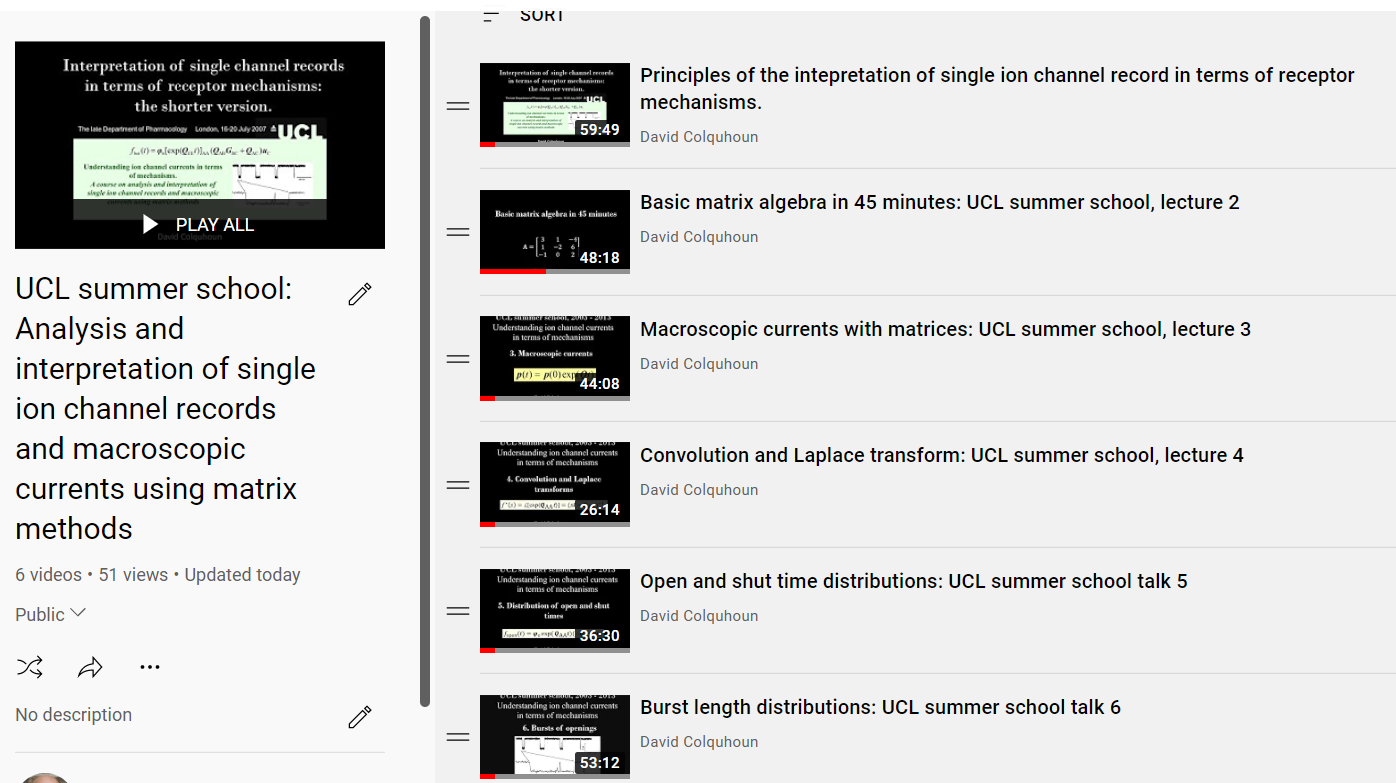### Workshop Description

A workshop on analysis and interpretation of single ion channel records and
macroscopic currents using matrix methods.

Aim of the workshop

The aim of this workshop is to explain the theoretical background that is needed to interpret ion channel experiments in terms of physical receptor mechanisms.
In order to do this, in any case apart from the very simplest, it is essential to know some basic ideas about matrices, and the workshop explains that (see lecture 2 in the playlist).

### The syllabus

(a) Introductory section for those with no prior knowledge of matrix algebra

1. Definition of a matrix
2. Addition, subtraction and multiplication of matrices. Commutation
3. Matrices as a convenient notation for simultaneous equations and as a convenient way of multiplying and adding probabilities, illustrated by a simple Markov chain.
4. Inversion of matrices, and definition of determinant.
5. Partitioned matrices
6. Characteristic polynomial of a matrix: eigenvalues and eigenvectors
7. Differentiation of a matrix and exponential of a matrix.

(b) Introductory section on statistics for those who need it

1. Continuous and discrete distributions
2. Probability density functions (the other meaning of ‘pdf’) and cumulative distribution functions
3. Rate constants, probabilities and derivation of simple exponential distribution
4. Convolution: meaning, done explicitly, and by Laplace transform method.
5. Description of macroscopic (‘whole-cell) currents in matrix notation: a single equation for any sort of experiment.
6. A simple ion channel mechanism analysed without matrices.

(c) Single ion channel analysis

1. Distributions of open and shut times using matrices -comparison with the same simple case as done above without matrices.
2. Division of single channel records into bursts and clusters of channel openings.
3. Number of openings per burst: simple case, geometric distribution.
4. Generalisation: sets of states and why matrices are useful; definition of Q matrix, and its partitioned forms.
5. Generalisation of analysis of bursts of openings.
6. What are the ubiquitous GAB and GAB(t) matrices?
7. The distribution of the number of openings per burst: general form.
8. The distribution of the open time, burst length and open time per burst; general forms.
9. Equilibrium occupancies and macroscopic currents.
10. The crucial role of entry probabilities: how to define the initial vectors for openings, $\varphi_o$, and for bursts, $\varphi_b$
11. How to calculate things like exp(QAAt): eigenvalues, eigenvectors and the beautiful spectral expansion theorem.
12. Correlations between open times and shut times (and the rank of a matrix). Bivariate and conditional distributions. Implications for connectivity of reaction schemes.
13. Single channels after a jump. Non-stationary single channel behaviour.
14. The relationship between single channel behaviour and macroscopic currents.

(d) Analysis and fitting of single channel data:

1. Use of programs for analysis of experimental data, particularly direct fitting of a mechanism with HJCFIT.

By the end of the workshop you should be able to understand the formula for the burst length distribution that appears on the workshop mug, at least well enough to evaluate it, and at best to be able to derive it.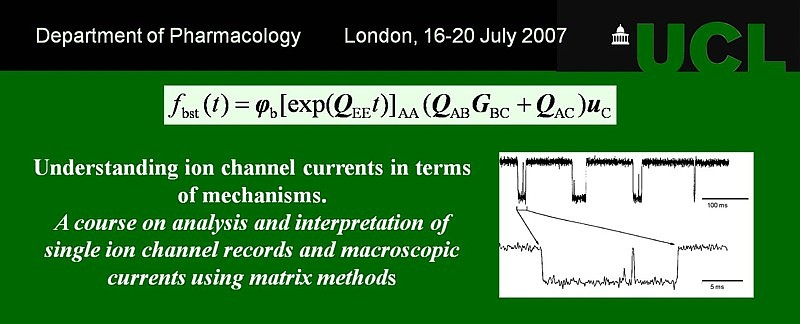(Note: in 2007 there was still a Department of Pharmacology at UCL)

### Workshop Organisers

• Workshop Directors Prof David Colquhoun and Prof Lucia Sivilotti (Pharmacology)
• Tutors:  Remigijus Lape and Andrew Plested.

### Course photographs

2013 course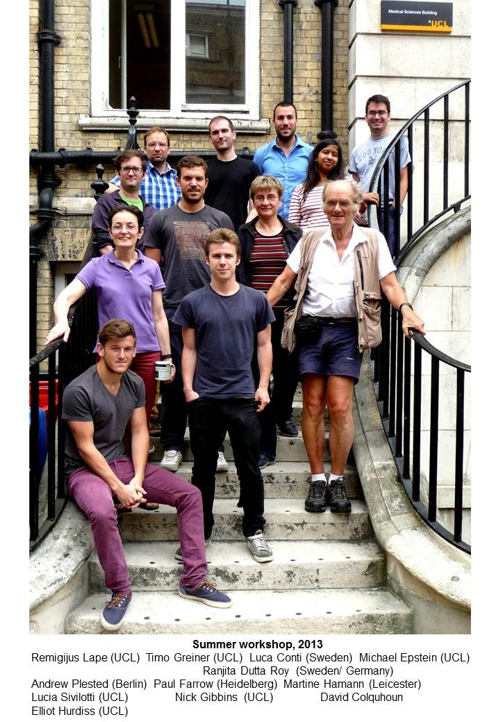2012 course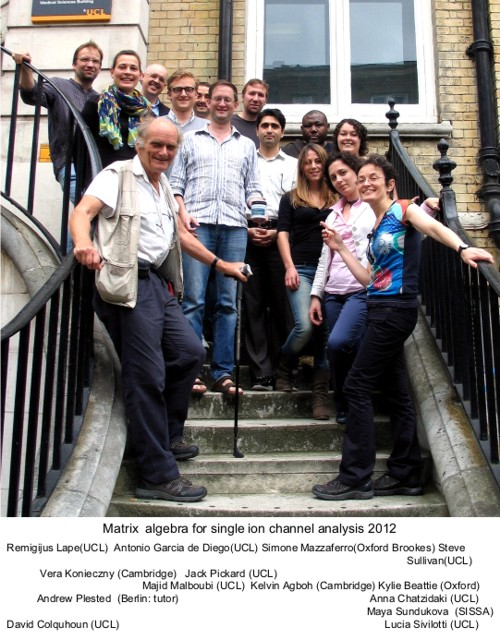2011 course